# 房室化神经元Chay模型的放电节律研究Firing Rhythm of Compartmentalized Neuron Chay Model

• 全文下载: PDF(838KB)    PP.204-219   DOI: 10.12677/AAM.2020.92024
• 下载量: 56  浏览量: 84

In this paper, taking the geometric morphology and structure of auditory neurons as the criteria of compartment division, two-compartment neuron Chay model and multi-compartment neuron Chay model were constructed. Then, the nonlinear dynamics analysis and neural computing of compart-mentalized neuron Chay model were carried out: 1) Two-compartment neuron Chay model. We the-oretically analyzed the periodic firing, as well as bifurcation sequence structures such as period adding bifurcation and period doubling bifurcation under the change of electrophysiological pa-rameters, meanwhile two kinds of bursting patterns and their transition mechanisms were studied by using the fast-slow dynamics. 2) Fifteen-compartment neuron Chay model. The stable transmis-sion of biological information, the directivity of external stimulation sites as well as the conse-quences of demyelination were numerically simulated. 3) One hundred and thirty-one-compartment neuron Chay model. They were investigated respectively that consisted of the effects of endogenic and extrinsic variable parameters as well as the geometric structure of the central axon on the rhythmic encoding methods such as the first spike latency, firing rate, maximum amplitude, and global synchronization. When the compartment models were used for nonlinear dynamic analysis including bifurcation, chaos and neural computing including information coding, the information transmission characteristics and degeneration mechanism of auditory neurons were verified and explained by numerical results in response to experimental conclusions and physiological phenomena.

1. 引言

2. 模型描述

2.1. 神经元Chay模型

1983年，Chay和Keizer提出了多尺度神经元Chay模型，展示了在静息状态和反复放电状态之间转迁的丰富簇放电模式，其中，不依赖电位而依赖Ca2+离子浓度的K+离子通道是很多可兴奋性细胞的重要特征，因此该模型常用来描述神经元、感觉末梢、心肌细胞等，模型描述如下：

$\left\{\begin{array}{l}{V}^{\prime }={g}_{I}{m}_{\infty }^{3}{h}_{\infty }\left({V}_{I}-V\right)+{g}_{KV}{n}^{4}\left({V}_{K}-V\right)+{g}_{KCa}C\left({V}_{K}-V\right)/\left(1+C\right)+{g}_{l}\left({V}_{l}-V\right),\text{ }\text{ }\text{ }\text{ }\text{ }\text{ }\text{ }\text{ }\text{ }\text{ }\text{ }\text{ }\text{ }\text{ }\text{ }\text{ }\text{ }\text{ }\text{ }\text{ }\text{ }\text{ }\text{ }\text{ }\text{ }\text{ }\text{ }\text{ }\text{ }\text{ }\text{ }\text{ }\text{ }\text{ }\text{ }\text{ }\text{ }\text{ }\text{ }\text{ }\text{ }\text{ }\text{ }\text{ }\text{ }\text{ }\text{ }\text{ }\text{ }\text{ }\text{ }\text{ }\text{ }\text{ }\text{ }\text{ }\text{ }\text{ }\text{ }\text{ }\text{ }\text{ }\text{ }\text{ }\text{ }\text{ }\text{ }\text{ }\left(1\right)\\ {n}^{\prime }=\left({n}_{\infty }-n\right)/{\tau }_{n},\text{ }\text{ }\text{ }\text{ }\text{ }\text{ }\text{ }\text{ }\text{ }\text{ }\text{ }\text{ }\text{ }\text{ }\text{ }\text{ }\text{ }\text{ }\text{ }\text{ }\text{ }\text{ }\text{ }\text{ }\text{ }\text{ }\text{ }\text{ }\text{ }\text{ }\text{ }\text{ }\text{ }\text{ }\text{ }\text{ }\text{ }\text{ }\text{ }\text{ }\text{ }\text{ }\text{ }\text{ }\text{ }\text{ }\text{ }\text{ }\text{ }\text{ }\text{ }\text{ }\text{ }\text{ }\text{ }\text{ }\text{ }\text{ }\text{ }\text{ }\text{ }\text{ }\text{ }\text{ }\text{ }\text{ }\text{ }\text{ }\text{ }\text{ }\text{ }\text{ }\text{ }\text{ }\text{ }\text{ }\text{ }\text{ }\text{ }\text{ }\text{ }\text{ }\text{ }\text{ }\text{ }\text{ }\text{ }\text{ }\text{ }\text{ }\text{ }\text{ }\text{ }\text{ }\text{ }\text{ }\text{ }\text{ }\text{ }\text{ }\text{ }\text{ }\text{ }\text{ }\text{ }\text{ }\text{ }\text{ }\text{ }\text{ }\text{ }\text{ }\text{ }\text{ }\text{ }\text{ }\text{ }\text{ }\text{ }\text{ }\text{ }\text{ }\text{ }\text{ }\text{ }\text{ }\text{ }\text{ }\text{ }\text{ }\text{ }\text{ }\text{ }\text{ }\text{ }\text{ }\text{ }\text{ }\text{ }\text{ }\text{ }\text{ }\text{ }\text{ }\text{ }\text{ }\text{ }\text{ }\text{ }\text{ }\text{ }\text{ }\text{ }\text{ }\text{ }\text{ }\text{ }\text{ }\text{ }\text{ }\text{ }\text{ }\text{ }\text{ }\text{ }\text{ }\text{ }\text{ }\text{ }\text{ }\text{ }\text{ }\text{ }\text{ }\text{ }\text{ }\text{ }\text{ }\text{ }\text{ }\text{ }\text{ }\text{ }\text{ }\text{ }\text{ }\text{ }\text{ }\text{ }\text{ }\text{ }\text{ }\text{ }\text{ }\text{ }\text{ }\text{ }\text{ }\text{ }\text{ }\text{ }\text{ }\text{ }\text{ }\text{ }\text{ }\text{ }\text{ }\text{ }\text{ }\text{ }\text{ }\text{ }\text{ }\text{ }\text{ }\text{ }\text{ }\text{ }\text{ }\text{ }\text{ }\text{ }\text{ }\text{ }\text{ }\text{ }\text{ }\text{ }\text{ }\text{ }\text{ }\text{ }\text{ }\text{ }\text{ }\text{ }\text{ }\text{ }\text{ }\text{ }\text{ }\text{ }\text{ }\text{ }\text{ }\text{ }\text{ }\text{ }\text{ }\text{ }\text{ }\text{ }\text{ }\text{ }\text{ }\text{ }\text{ }\text{ }\text{ }\text{ }\text{ }\text{ }\text{ }\text{ }\text{ }\text{ }\text{ }\text{ }\text{ }\text{ }\text{ }\text{ }\text{ }\text{ }\text{ }\text{ }\text{ }\text{ }\text{ }\text{ }\text{ }\text{ }\text{ }\text{ }\text{ }\text{ }\text{ }\text{ }\text{ }\text{ }\text{ }\text{ }\text{ }\text{ }\text{ }\text{ }\text{ }\text{ }\text{ }\text{ }\text{ }\text{ }\text{ }\text{ }\text{ }\text{ }\text{ }\text{ }\left(2\right)\\ {C}^{\prime }=\rho \left[{m}_{\infty }^{3}{h}_{\infty }\left({V}_{C}-V\right)-{k}_{C}C\right]\text{ }\text{ }\text{ }\text{ }\text{ }\text{ }\text{ }\text{ }\text{ }\text{ }\text{ }\text{ }\text{ }\text{ }\text{ }\text{ }\text{ }\text{ }\text{ }\text{ }\text{ }\text{ }\text{ }\text{ }\text{ }\text{ }\text{ }\text{ }\text{ }\text{ }\text{ }\text{ }\text{ }\text{ }\text{ }\text{ }\text{ }\text{ }\text{ }\text{ }\text{ }\text{ }\text{ }\text{ }\text{ }\text{ }\text{ }\text{ }\text{ }\text{ }\text{ }\text{ }\text{ }\text{ }\text{ }\text{ }\text{ }\text{ }\text{ }\text{ }\text{ }\text{ }\text{ }\text{ }\text{ }\text{ }\text{ }\text{ }\text{ }\text{ }\text{ }\text{ }\text{ }\text{ }\text{ }\text{ }\text{ }\text{ }\text{ }\text{ }\text{ }\text{ }\text{ }\text{ }\text{ }\text{ }\text{ }\text{ }\text{ }\text{ }\text{ }\text{ }\text{ }\text{ }\text{ }\text{ }\text{ }\text{ }\text{ }\text{ }\text{ }\text{ }\text{ }\text{ }\text{ }\text{ }\text{ }\text{ }\text{ }\text{ }\text{ }\text{ }\text{ }\text{ }\text{ }\text{ }\text{ }\text{ }\text{ }\text{ }\text{ }\text{ }\text{ }\text{ }\text{ }\text{ }\text{ }\text{ }\text{ }\text{ }\text{ }\text{ }\text{ }\text{ }\text{ }\text{ }\text{ }\text{ }\text{ }\text{ }\text{ }\text{ }\text{ }\text{ }\text{ }\text{ }\text{ }\text{ }\text{ }\text{ }\text{ }\text{ }\text{ }\text{ }\text{ }\text{ }\text{ }\text{ }\text{ }\text{ }\text{ }\text{ }\text{ }\text{ }\text{ }\text{ }\text{ }\text{ }\text{ }\text{ }\text{ }\text{ }\text{ }\text{ }\text{ }\text{ }\text{ }\text{ }\text{ }\text{ }\text{ }\text{ }\text{ }\text{ }\text{ }\text{ }\text{ }\text{ }\text{ }\text{ }\text{ }\text{ }\text{ }\text{ }\text{ }\text{ }\text{ }\text{ }\text{ }\text{ }\text{ }\text{ }\text{ }\text{ }\text{ }\text{ }\text{ }\text{ }\text{ }\text{ }\text{ }\text{ }\text{ }\text{ }\text{ }\text{ }\text{ }\text{ }\text{ }\text{ }\text{ }\text{ }\text{ }\text{ }\text{ }\text{ }\text{ }\text{ }\text{ }\text{ }\text{ }\text{ }\text{ }\text{ }\text{ }\text{ }\text{ }\text{ }\text{ }\text{ }\text{ }\text{ }\text{ }\text{ }\text{ }\text{ }\text{ }\text{ }\text{ }\text{ }\text{ }\text{ }\text{ }\text{ }\text{ }\text{ }\text{ }\text{ }\left( 3 \right)\end{array}$

(1)式表示神经元膜电位V的变化速率，右边分别是混合Na+-Ca2+离子通道电流、依赖于电位的K+离子通道电流、不依赖电位而依赖Ca2+离子浓度的K+离子通道电流和漏电流。(2)式为依赖于电位的K+离子通道打开概率n的变化速率。(3)式则为Ca2+离子浓度C的变化速率。其中， ${m}_{\infty }$${h}_{\infty }$ 分别是混合Na+-Ca2+离子通道激活和失活概率的稳态值， ${g}_{I}$${g}_{KV}$${g}_{KCa}$${g}_{l}$ 分别代表各离子通道的最大电导， ${V}_{I}$${V}_{KV}$${V}_{KCa}$${V}_{l}$${V}_{C}$ 为各离子通道的平衡电位， ${n}_{\infty }$ 是n的稳态值， ${\tau }_{n}$ 为弛豫时间， $\rho$ 是比例常数， ${k}_{C}$ 是细胞内Ca2+离子流出的比率常数。并且

${x}_{\infty }=\frac{{\alpha }_{x}}{{\alpha }_{x}+{\beta }_{x}},\left(x=m,n,h\right)$

$\begin{array}{l}{\alpha }_{m}=\frac{0.1\left(25+V\right)}{1-\mathrm{exp}\left(-0.1V-2.5\right)},\text{ }\text{ }\text{ }\text{ }\text{ }\text{ }\text{ }\text{ }\text{ }\text{ }\text{ }\text{ }\text{ }\text{ }\text{ }\text{ }\text{ }\text{ }\text{ }\text{ }\text{ }\text{ }\text{ }\text{ }\text{ }\text{ }\text{ }\text{ }\text{ }\text{ }\text{ }\text{ }\text{ }\text{ }\text{ }\text{ }\text{ }\text{ }\text{ }\text{ }\text{ }\text{ }\text{ }\text{ }\text{ }\text{ }\text{ }\text{ }\text{ }\text{ }{\beta }_{m}=4\mathrm{exp}\left(-\frac{V+50}{18}\right),\\ {\alpha }_{h}=0.07\mathrm{exp}\left(-0.05V-2.5\right),\text{ }\text{ }\text{ }\text{ }\text{ }\text{ }\text{ }\text{ }\text{ }\text{ }\text{ }\text{ }\text{ }\text{ }\text{ }\text{ }\text{ }\text{ }\text{ }\text{ }\text{ }\text{ }\text{ }\text{ }\text{ }\text{ }\text{ }\text{ }\text{ }\text{ }\text{ }\text{ }\text{ }\text{ }\text{ }\text{ }\text{ }\text{ }\text{ }\text{ }\text{ }{\beta }_{h}=\frac{1}{1+\mathrm{exp}\left(-0.1V-2\right)},\\ {\alpha }_{n}=\frac{0.01\left(20+V\right)}{1-\mathrm{exp}\left(-0.1V-2\right)},\text{ }\text{ }\text{ }\text{ }\text{ }\text{ }\text{ }\text{ }\text{ }\text{ }\text{ }\text{ }\text{ }\text{ }\text{ }\text{ }\text{ }\text{ }\text{ }\text{ }\text{ }\text{ }\text{ }\text{ }\text{ }\text{ }\text{ }\text{ }\text{ }\text{ }\text{ }\text{ }\text{ }\text{ }\text{ }\text{ }\text{ }\text{ }\text{ }\text{ }\text{ }\text{ }\text{ }\text{ }\text{ }\text{ }\text{ }\text{ }\text{ }\text{ }\text{ }\text{ }\text{ }\text{ }\text{ }\text{ }\text{ }\text{ }\text{ }{\beta }_{n}=0.125\mathrm{exp}\left(-\frac{V+30}{80}\right),\\ {\tau }_{n}=\frac{1}{{\lambda }_{n}\left({\alpha }_{n}+{\beta }_{n}\right)}.\end{array}$

2.2. 听神经元的模型描述

$\frac{\text{d}{V}_{i+1}}{\text{d}t}=-{g}_{int}{V}_{i+1}/{C}_{m,i+1}$

$\begin{array}{l}{C}_{m,i+1}={\left(\frac{1}{{C}_{mem}}+\frac{{N}_{my}}{{C}_{my}}\right)}^{-1}\\ {g}_{int}=\frac{1}{\left({N}_{my}{R}_{my}\right)+{R}_{mem}}\end{array}$

3. 两房室神经元Chay模型

${{V}^{\prime }}_{1}=-{I}_{ion,1}+{I}_{stim}+k\left({V}_{2}-{V}_{1}\right)/\left({R}_{2}/2+{R}_{1}/2\right)$ (4)

${{n}^{\prime }}_{1}=\left({n}_{\infty ,1}-{n}_{1}\right)/{\tau }_{n,1}$ (5)

${{V}^{\prime }}_{2}=-{I}_{ion,2}+k\left({V}_{1}-{V}_{2}\right)/\left({R}_{1}/2+{R}_{2}/2\right)$ (6)

${{n}^{\prime }}_{2}=\left({n}_{\infty ,2}-{n}_{2}\right)/{\tau }_{n,2}$ (7)

${{C}^{\prime }}_{1}=\rho \left[{m}_{\infty ,1}^{3}{h}_{\infty ,1}\left({V}_{C}-{V}_{1}\right)-{k}_{C}{C}_{1}\right]$ (8)

${{C}^{\prime }}_{2}=\rho \left[{m}_{\infty ,2}^{3}{h}_{\infty ,2}\left({V}_{C}-{V}_{2}\right)-{k}_{C}{C}_{2}\right]$ (9)

3.1. 参数的敏感性

3.1.1. 峰峰间距和簇簇间距

3.1.2. 峰相位差和簇相位差

$\Delta {\phi }_{s}\left(t\right)=2\pi \frac{{t}_{s}-{t}_{n}}{{t}_{n+1}-{t}_{n}},{t}_{n}<{t}_{s}\le {t}_{n+1}$

$\Delta {\phi }_{b}\left(t\right)=2\pi \frac{{\tau }_{s}-{\tau }_{n}}{{\tau }_{n+1}-{\tau }_{n}},{\tau }_{n}<{\tau }_{s}\le {\tau }_{n+1}$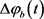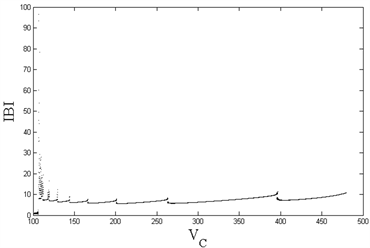Figure 1. (Top left): Changes in ISI of compartment 1 with VC; (Top right): Changes in IBI of compartment 1 with VC; (Bottom left): Changes in ISI of compartment 2 with VC; (Bottom right): Changes in IBI of compartment 2 with VC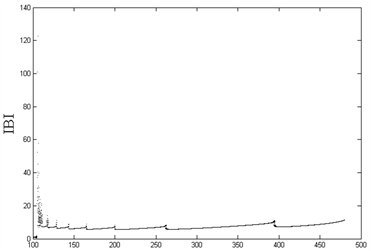Figure 2. (Left): Phase difference of spikes between compartment 1 and compartment 2; (Right): Phase difference of bursts between compartment 1 and compartment 2

3.2. 不同类型的簇放电模式及其转迁

3.2.1. 经由“fold/homoclinic”滞后环的“fold/homoclinic”型簇放电

${g}_{KV}=1350\text{\hspace{0.17em}}{\text{s}}^{-1},{V}_{K}=-75\text{\hspace{0.17em}}\text{mV}$ 时，系统(4)~(9)中房室1的放电模式如图3(左)膜电位的时间历程图，快子系统(4)~(7)的平衡点分岔曲线以慢变量 ${C}_{1}$ 作为分岔参数，并将两房室系统(4)~(9)的相平面图 $\left({C}_{1},{V}_{1}\right)$ 附加其上，得到图3(右)快慢动力学分岔图。

3.2.2. 经由“fold/homoclinic”滞后环的“Hopf/homoclinic”型簇放电

${g}_{KV}=1000\text{\hspace{0.17em}}{\text{s}}^{-1},\text{\hspace{0.17em}}{V}_{K}=-75\text{\hspace{0.17em}}\text{mV}$ 时，系统(4)~(9)中房室1的放电模式如图4(左)膜电位的时间历程图所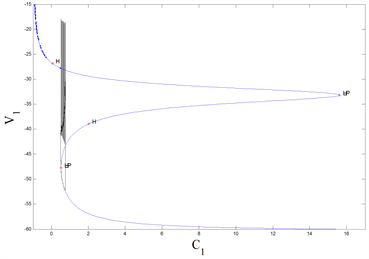Figure 3. The “fold/homoclinic” bursting via the “fold/homoclinic” hysteresis loop in the system (4)~(9) when ${g}_{KV}=1350\text{\hspace{0.17em}}{\text{s}}^{-1},\text{\hspace{0.17em}}{V}_{K}=-75\text{\hspace{0.17em}}\text{mV}$. (Left): Time series of the membrane potential; (Right): Fast-slow dynamics bifurcation diagramFigure 4. The “Hopf/homoclinic” bursting via the “fold/homoclinic” hysteresis loop in the system (4)~(9) when ${g}_{KV}=1000\text{\hspace{0.17em}}{\text{s}}^{-1},\text{\hspace{0.17em}}{V}_{K}=-75\text{\hspace{0.17em}}\text{mV}$. (Left): Time series of the membrane potential; (Right): Fast-slow dynamics bifurcation diagram

4. 多房室神经元Chay模型

4.1. 15房室神经元Chay模型

I型听神经元占听神经元总数的90%以上，有髓鞘，而II型听神经元的占比不到10%，无髓鞘。因此，运用理论模型对I、II型听神经元数值模拟，可以研究不同听神经元信息传递的特点以及节律编码的丰富模式。如第2章所言，听神经元未包裹髓鞘的房室用Chay模型描述，包裹髓鞘的房室则为双电缆结构，据此，基于I型听神经元  的形态结构，构建15房室神经元Chay模型，研究信息传递特点、刺激位点搭配以及退化机制等生理功能规律。

4.1.1. 两类听神经元放电模式的比较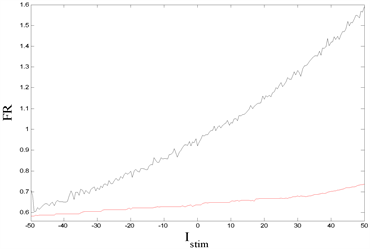Figure 5. (Left): First spike latency (FSL) of two kinds of auditory neurons when ${I}_{stim}=-50~50\text{\hspace{0.17em}}\text{mA}$ ; (Right): Firing rate (FR) of two kinds of auditory neurons when ${I}_{stim}=-50~50\text{\hspace{0.17em}}\text{mA}$. Red: Type I auditory neuron. Black: Type II auditory neuron

4.1.2. 外界刺激对不同房室的影响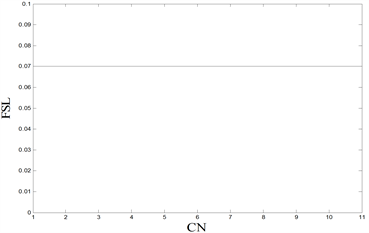Figure 6. (Left): The first spike of Ranvier nodes and soma at Istim = 30 mA; (Right): The first spike of Ranvier nodes and soma at Istim = 30 mA. From top to bottom: compartment 1-compartment 3- ... -compartment 11-compartment 12 (soma)-compartment 13-compartment 15. For the convenience of observation, each compartment in the figures is shifted down by 2 units in the vertical direction from the previous compartment, and the soma is shown in red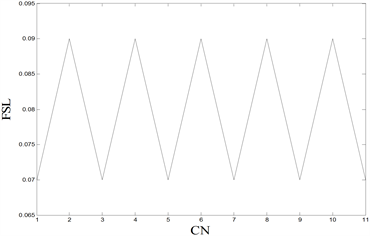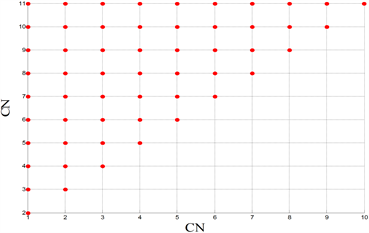Figure 7. Comparisons of FSL under different stimulations. (Top left): Only one compartment is stimulated, and the abscissa is the compartment number (CN) for current stimulation. (Top right): Two compartments are stimulated at the same time. The abscissa and ordinate are the compartment number (CN) stimulated by the current. The FSL of the red dot is 0.07 s, and the FSL of the blue dot is 0.09 s. (Bottom left): Nine compartments are stimulated at the same time. The abscissa and ordinate are the compartment number (CN) without current stimulation, and the FSL of the red dot is 0.07 s. (Bottom right): Ten compartments are stimulated at the same time, and the abscissa is the compartment number (CN) without current stimulation

4.1.3. 听神经元的退化模拟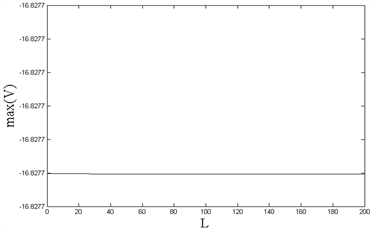Figure 8. (Left): Changes of FSL with L (length of demyelination); (Right): Changes of the maximum amplitude of the soma with L (length of demyelination)

4.2. 131房室神经元Chay模型

4.2.1. 不同参数的敏感性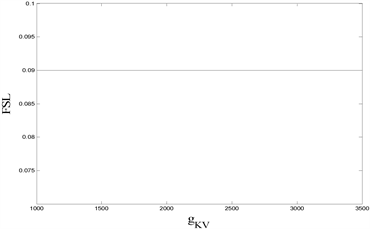Figure 9. (Left): Changes of FSL with ${I}_{steam}$. (Right): Changes of FSLwith ${g}_{KV}$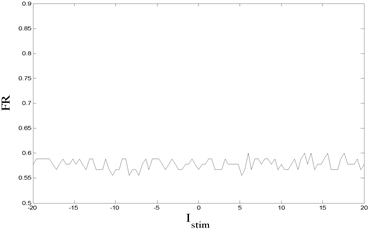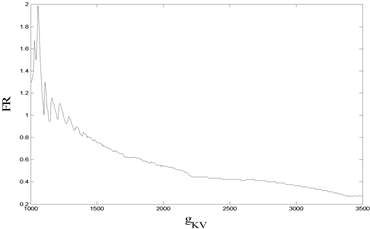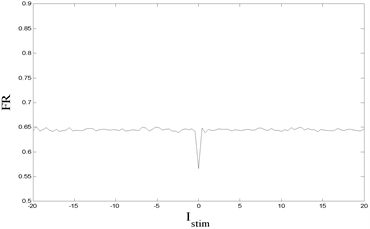Figure 10. Changes of FR in various parts of neurons with parameters. (Top left): $\text{FR}-{I}_{stim}$ diagram of the soma; (Top right): $\text{FR}-{g}_{KV}$ diagram of the soma; (Bottom left): $\text{FR}-{I}_{stim}$ diagram of the terminal button of the central axon; (Bottom right): $\text{FR}-{g}_{KV}$ diagram of the terminal button of the central axon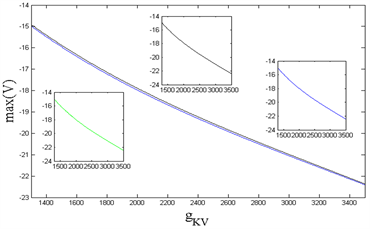Figure 11. (Left): The variation of maximum amplitude of compartment 1, 2, and 6 with Istim; (Right): The variation of maximum amplitude of compartment 1, 2, and 6 with gKV. Among them, black: compartment 1, green: compartment 2, blue: compartment 6, and in the two figures, the red and green curves partially overlap due to similar values

4.2.2. 全局同步性

$e\left(t\right)=\frac{\underset{i\ne 1}{\sum }\left({\left({V}_{i}-{V}_{1}\right)}^{2}+{\left({n}_{i}-{n}_{1}\right)}^{2}+{\left({C}_{i}-{C}_{1}\right)}^{2}\right)}{N-1}$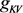Figure 12. Changes of maximum synchronization difference caused by different parameters. (Left): ${I}_{stim}-{V}_{K}-\mathrm{max}\left(e\right)$ diagram; (Right): ${V}_{C}-{g}_{KV}-\mathrm{max}\left(e\right)$ diagram

4.2.3. 中枢突结构对放电模式的影响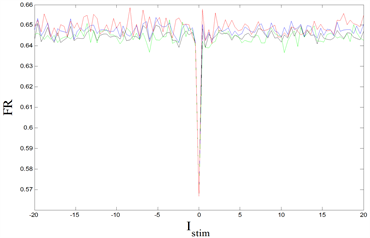Figure 13. (Left): FR − Istim diagram of the terminal button of the central axon in four cases; (Right): FR − gKV diagram of the terminal button of the central axon in four cases. Among them, black: normal case, green: remove parts of central axon corresponding to AVCN, blue: remove part of central axon corresponding to DCN, red: remove part of central axon corresponding to PVCN

5. 讨论

  Hodgkin, A.L. and Huxley, A.F. (1952) A Quantitative Description of Membrane Current and Its Application to Con-duction and Excitation in Nerve. The Journal of Physiology, 117, 500-544. https://doi.org/10.1113/jphysiol.1952.sp004764  Chay, T.R. and Keizer, J. (1983) Minimal Model for Mem-brane Oscillations in the Pancreatic Beta-Cell. Biophysical Journal, 42, 181-189. https://doi.org/10.1016/S0006-3495(83)84384-7  Lisman, J.E. (1997) Bursts as a Unit of Neural Information: Making Unreliable Synapses Reliable. Trends in Neurosciences, 20, 38-43. https://doi.org/10.1016/S0166-2236(96)10070-9  Rinzel, J. and Ermentrout, G.B. (1989) Analysis of Neural Excitability and Oscillations. In: Koch, C. and Segev, I., Eds., Methods in Neuronal Modeling, MIT Press, Cambridge, 135-169.  Bertram, R., Butte, M.J., Kiemel, T. and Sherman, A. (1995) Topological and Phenomenological Clas-sification of Bursting Oscillations. Bulletin of Mathematical Biology, 57, 413-439. https://doi.org/10.1016/S0092-8240(05)81776-8  Izhikevich, E.M. (2000) Neural Excitability, Spiking and Bursting. International Journal of Bifurcation and Chaos, 10, 1171-1266. https://doi.org/10.1142/S0218127400000840  Ermentrout, G.B. and Terman, D.H. (2010) Mathematical Foundations of Neuroscience. Springer, Berlin, 117. https://doi.org/10.1007/978-0-387-87708-2  Wang, H.Y., Wang, J., Li, H.L., Chen, Y.Y. and Wei, X.L. (2012) Parameters Estimation and Dynamics Control of Chay Model Using UKF. Advanced Materials Research, 462, 693-700. https://doi.org/10.4028/www.scientific.net/AMR.462.693  裴利军, 王永刚, 范烨. 神经元Chay模型的动力学分析[J]. 郑州大学学报(理学版), 2009, 41(2): 7-12.  杨卓琴, 陆启韶. 神经元Chay模型中不同类型的簇放电模式[J]. 中国科学(G辑: 物理学 力学 天文学), 2007, 37(4): 440-450.  Duan, L.X. and Lu, Q.S. (2006) Codimension-Two Bifurcation Analysis on Firing Activities in Chay Neuron Model. Chaos Solitons & Fractals, 30, 1172-1179. https://doi.org/10.1016/j.chaos.2005.08.179  Duan, L.X., Lu, Q.S. and Wang, Q.Y. (2008) Two-Parameter Bifurcation Analysis of Firing Activities in the Chay Neuronal Model. Neurocomputing, 72, 341-351. https://doi.org/10.1016/j.neucom.2008.01.019  古华光, 任维, 陆启韶, 杨明浩. 实验性神经起步点自发放电的分叉和整数倍节律[J]. 生物物理学报, 2001, 17(4): 637-644.  王青云, 陆启韶. 噪声在慢变系统中的随机Chay神经元模型的自共振[J]. 动力学与控制学报, 2004, 2(3): 87-91.  Brown, M.C., Berglund, A.M., Kiang, N.Y. and Ryugo, D.K. (1988) Central Trajectories of Type II Spiral Ganglion Neurons. The Journal of Comparative Neurology, 278, 581-590. https://doi.org/10.1002/cne.902780409  Nayagam, B.A., Muniak, M.A. and Ryugo, D.K. (2011) The Spiral Ganglion: Connecting the Peripheral and Central Auditory Systems. Hearing Research, 278, 2-20. https://doi.org/10.1016/j.heares.2011.04.003  Berglund, A.M. and Brown, M.C. (1994) Central Trajec-tories of Type II Spiral Ganglion Cells from Various Cochlear Regions in Mice. Hearing Research, 75, 121-130. https://doi.org/10.1016/0378-5955(94)90063-9  Blight, A.R. (1985) Computer Simulation of Action Potentials and Afterpotentials in Mammalian Myelinated Axons: The Case for a Lower Resistance Myelin Sheath. Neuroscience, 15, 13-31. https://doi.org/10.1016/0306-4522(85)90119-8  Smit, J.E., Hanekom, T.H. and Hanekom, J.J. (2008) Pre-dicting Action Potential Characteristics of Human Auditory Nerve Fibres through Modification of the Hodgkin-Huxley Equations. South African Journal of Science, 104, 284-292.  Wang, H.X., Lu, Q.S. and Shi, X. (2010) Phase Syn-chronization and Its Transition in Two Coupled Bursting Neurons: Theoretical and Numerical Analysis. Chinese Physics B, 19, Article ID: 060509. https://doi.org/10.1088/1674-1056/19/6/060509  Rinzel, J. (1985) Bursting Oscillations in an Excitable Membrane Model. In: Sleeman, B.D. and Jarvis, R.J., Eds., Ordinary and Partial Differential Equations, Springer, New York, 304-316. https://doi.org/10.1007/BFb0074739  Rattay, F., Lutter, P. and Felix, H. (2001) A Model of the Electrically Excited Human Cochlear Neuron I. Contribution of Neural Substructures to the Generation and Propagation of Spikes. Hearing Research, 153, 43-63. https://doi.org/10.1016/S0378-5955(00)00256-2  Miller, C.A., Abbas, P.J., Robinson, B.K., Rubinstein, J.T. and Matsuoka, A.J. (1999) Electrically Evoked Single-Fiber Action Potentials from Cat: Responses to Monopolar, Monophasic Stimulation. Hearing Research, 130, 197-218. https://doi.org/10.1016/S0378-5955(99)00012-X  Rubinstein, J.T. (1991) Analytical Theory for Extracellular Electrical Stimulation of Nerve with Focal Electrodes. II. Passive Myelinated Axon. Biophysical Journal, 60, 538-555. https://doi.org/10.1016/S0006-3495(91)82084-7  Briaire, J.J. and Frijns, J.H. (2006) The Consequences of Neural Degeneration Regarding Optimal Cochlear Implant Position in Scala Tympani: A Model Approach. Hearing Research, 214, 17-27. https://doi.org/10.1016/j.heares.2006.01.015  Schuknecht, H.F. (1993) Pathology of the Ear, 2nd Edition, Lea and Febiger, Philadelphia.  温娜. 规则连接神经网络的同步分析与模拟[D]: [硕士学位论文]. 广州: 华南理工大学, 2019.